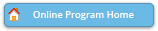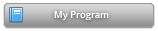#### Abstract Details

 Activity Number: 255 - Contributed Poster Presentations: Section on Statistical Computing Type: Contributed Date/Time: Monday, July 29, 2019 : 2:00 PM to 3:50 PM Sponsor: Section on Statistical Computing Abstract #306724 Title: Autocorrelation Function Estimation Using Penalized Least Squares Author(s): Xiyan Tan* and Colin Mark Gallagher Companies: Clemson University and Clemson University Keywords: Autocorrelation function; Penalized Regression; Time series analysis; Estimation bias; Tuning parameter selection; Standard error Abstract: It has long been known that the sample autocorrelation underestimates the magnitude of autocorrelation in stationary time series. Finite sample bias corrections can be found under specific assumed models. We consider model independent estimation of autocorrelation using penalized least squares. Unlike the regression case, in which the least squares estimator is unbiased and shrinkage is used to reduce mean squared error by introducing bias, in the autocorrelation case the usual estimator has bias toward zero. We choose penalties that can simultaneously reduce both bias and mean squared error when the magnitude of the correlation is high. The penalty can be chosen so that the resulting estimator of autocorrelation is non-negative definite and asymptotically normally distributed. A similar idea can be applied in estimating partial correlation. The resulting estimators can provide better estimates of standard error in time series regression models, and can improve power in time series diagnostic tests. The performance of the proposed methods is demonstrated on simulated data and applied to example data.

Authors who are presenting talks have a * after their name.